Premium

# 2.NBT.A.1 Worksheets, Workbooks, Lesson Plans, and Games

#### CCSS.maths.CONTENT.2.NBT.A.1

:
"Understand that the three digits of a three-digit number represent amounts of hundreds, tens, and ones; e.g., 706 equals 7 hundreds, 0 tens, and 6 ones. Understand the following as special cases:"

Our Take:Second graders should be expanding their place value knowledge, understanding that 10 tens grouped together is called a “hundred.” Help students learn, for example, that a three-digit number is composed of a ones, tens, and hundreds place. Second graders can illustrate this understanding by identifying the value of each digit in a number, as well as recording that number in the word and standard form.

These worksheets and lesson plans can help students practise this Common Core State Standards skill.

## Worksheets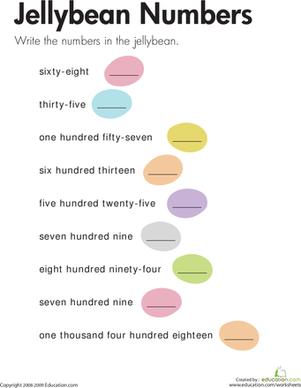Writing Numbers: Jellybean Numbers
Worksheet
Writing Numbers: Jellybean Numbers
On this year three maths worksheet, kids translate numbers written with words to numbers written with numerals up to four digits long.
Year 3
Maths
Worksheet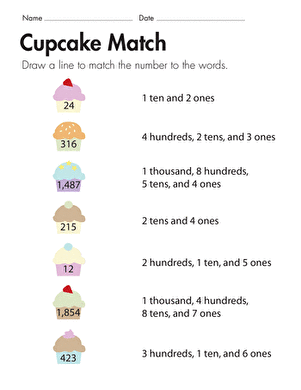Number Place Values: Cupcake Match
Worksheet
Number Place Values: Cupcake Match
Each yummy cupcake in this worksheet contains a number. In this place value maths worksheet, kids match each number to its written equivalent.
Year 3
Maths
Worksheet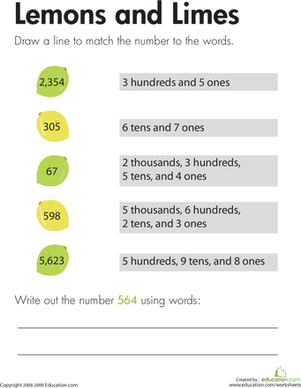Place Value: Lemons and Limes
Worksheet
Place Value: Lemons and Limes
Each sour fruit in this worksheet has a number written inside. Kids will match each number to the written equivalent of the number.
Year 3
Maths
Worksheet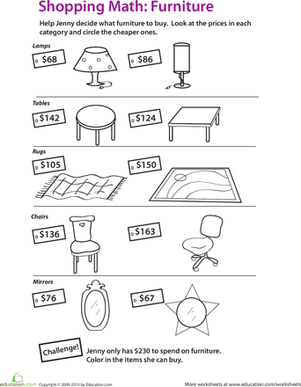Shopping with Place Value
Worksheet
Shopping with Place Value
In this year three maths worksheet, your child will learn about place value, practise reading whole numbers, and learn about shopping within a budget.
Year 3
Maths
Worksheet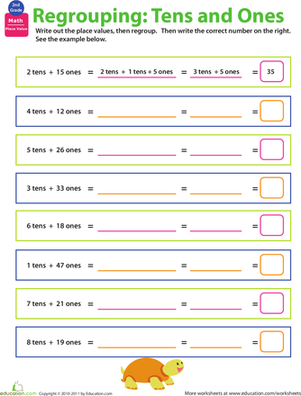Regrouping: practise Place Value #1
Worksheet
Regrouping: practise Place Value #1
This worksheet tests kids' understanding of place value by challenging them to regroup place values two different ways.
Year 3
Worksheet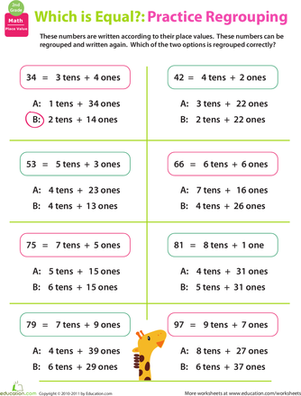Regrouping: practise Place Value #2
Worksheet
Regrouping: practise Place Value #2
Kids take on place value regrouping in this worksheet asks them to circle the place values that are regrouped correctly.
Year 3
Worksheet

## Lesson Plans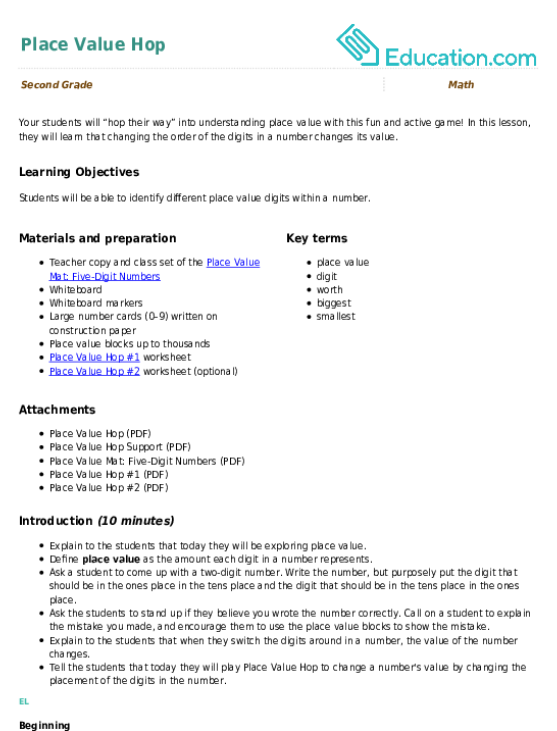Place Value Hop
Lesson plan
Place Value Hop
Your students will “hop their way” into understanding place value with this fun and active game! In this lesson, they will learn that changing the order of the digits in a number changes its value.
Year 3
Maths
Lesson planLet’s Compare! Place Value Disks & Three-Digit Numbers
Lesson plan
Let’s Compare! Place Value Disks & Three-Digit Numbers
Dive into understanding place value by using place value disks to represent and compare numbers.
Year 3
Maths
Lesson plan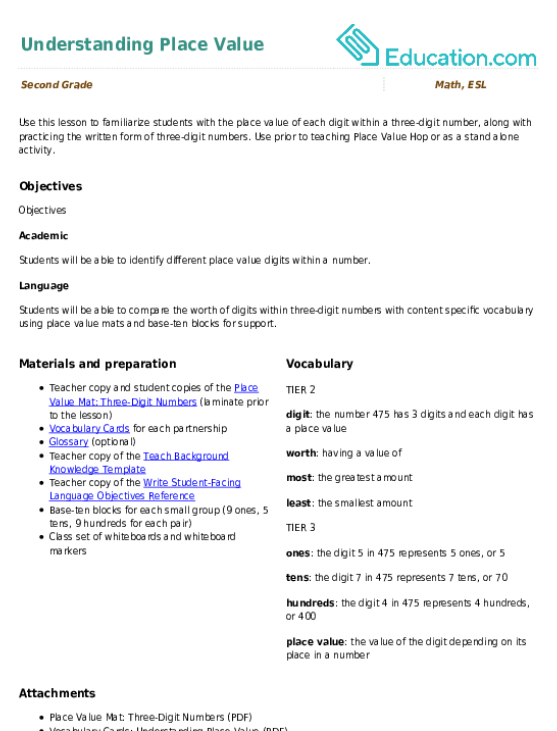Understanding Place Value
Lesson plan
Understanding Place Value
Use this lesson to familiarize students with the place value of each digit within a three-digit number, along with practising the written form of three-digit numbers. Use prior to teaching Place Value Hop or as a stand alone activity.
Year 3
Maths
Lesson planBase-Ten Blocks
Lesson plan
Base-Ten Blocks
Use this lesson to introduce the tool of base-ten blocks to your students. This scaffolded EL support lesson can be used as a stand-alone activity, or prior to teaching Three-Digit Addition with Base-Ten Models.
Year 3
Maths
Lesson plan

## Workbooks

No workbooks found for this common core node.

## Games

No games found for this common core node.

## Exercises

No exercises found for this common core node.

### Add to collection

Create new collection

0

### New Collection>

0Items

What could we do to improve Education.com?

Please note: Use the Contact Us link at the bottom of our website for account-specific questions or issues.

What would make you love Education.com?